# Supplementary Angles

Go back to  'Angles'

 1 What are Supplementary Angles? 2 Adjacent and Non-Adjacent Supplementary Angles (With Illustrations) 3 Tips and Tricks 4 How to Find Supplement of an Angle? (With an Activity) 5 Properties of Supplementary Angles 6 Supplementary Angle Theorem (with Illustration) 7 Solved Examples on Supplementary Angles 8 Challenging Questions on Supplementary Angles 9 Practice Questions on Supplementary Angles 10 Maths Olympiad Sample Papers 11 Frequently Asked Questions (FAQs)

We at Cuemath believe that Math is a life skill. Our Math Experts focus on the “Why” behind the “What.” Students can explore from a huge range of interactive worksheets, visuals, simulations, practice tests, and more to understand a concept in depth.

Book a FREE trial class today! and experience Cuemath's LIVE Online Class with your child.

## What are Supplementary Angles?

### Definition of Supplementary Angles

Two angles are said to be supplementary angles if they add up to 180 degrees.

The supplementary angles form a straight angle (180 degrees) when they are put together.

 $$\angle 1$$ and $$\angle 2$$ are supplementary if $\angle 1+ \angle 2 = 180^\circ$

In this case, $$\angle 1$$ and $$\angle 2$$ are called "supplements" of each other.

### Examples of Supplementary Angles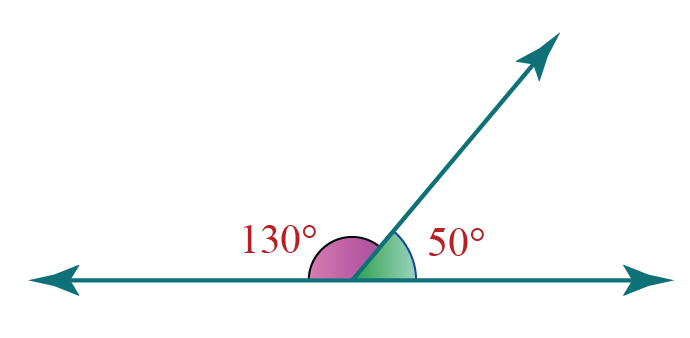In the above figure, $$130^\circ+50^\circ = 180^\circ$$

Hence, from the "Definition of Supplementary Angles", these two angles are supplementary.

Each angle among the supplementary angles is called the "supplement" of the other angle.

Here,

• 130o is the supplement of 50o

• 50o is the supplement of 130o

Some real-life examples of supplementary angles are as follows: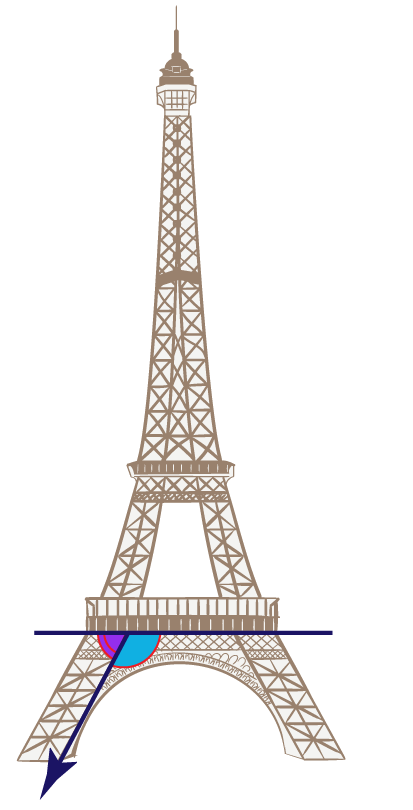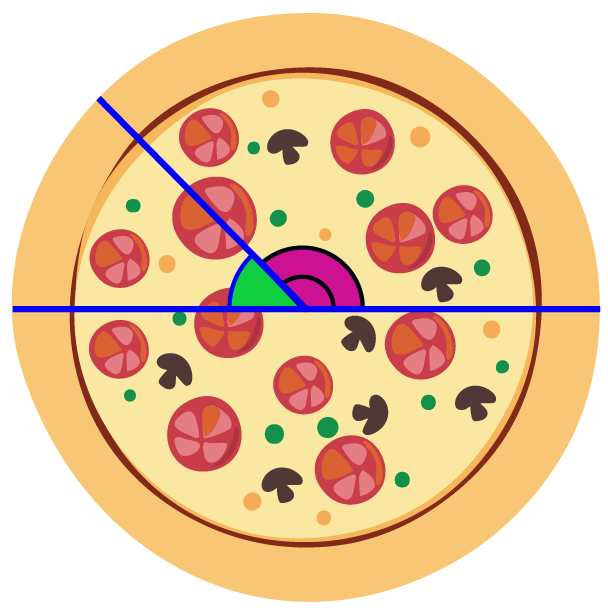The two angles in each of the above figures are adjacent (it means they have a common vertex and a common arm).

But do supplementary angles always need to be adjacent?

No, they can be non-adjacent as well.

Let us see how this is possible.

There are two types of supplementary angles.

Two supplementary angles with a common vertex and a common arm are said to be adjacent supplementary angles.

Example: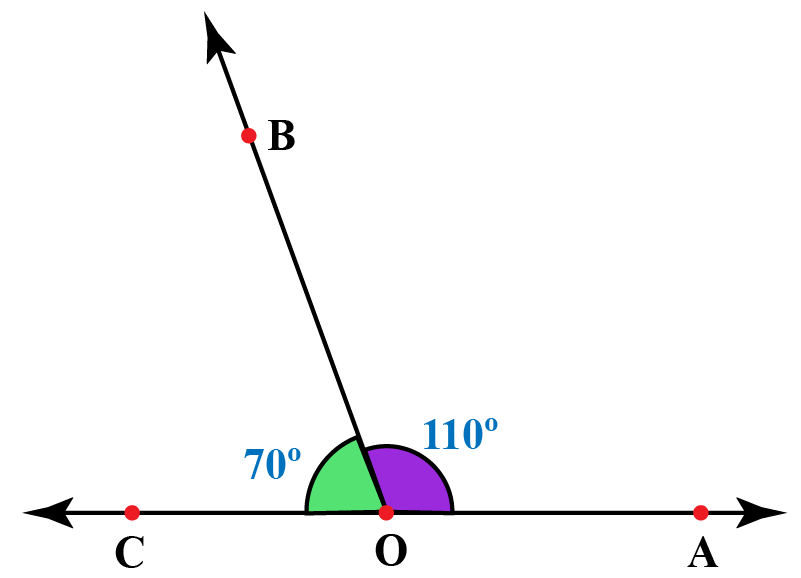Here, $$\angle COB$$ and $$\angle AOB$$ are adjacent angles as they have a common vertex, $$O$$, and a common arm $$OB$$

They also add up to 180 degrees. i.e.,

$\angle COB + \angle AOB = 70^\circ+110^\circ=180^\circ$

Hence, these two angles are adjacent supplementary angles.

You can observe the adjacent supplementary angles in the following illustration.

Move point C to change the angles and then click "GO"

Two supplementary angles that are NOT adjacent are said to be non-adjacent supplementary angles.

Example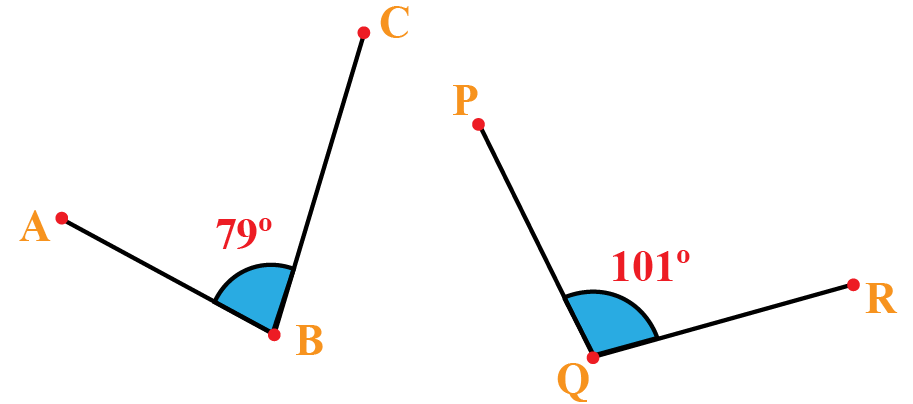Here, $$\angle ABC$$ and $$\angle PQR$$ are non-adjacent angles as they neither have a common vertex nor a common arm.

They also add up to 180 degrees. i.e.,

$\angle ABC+ \angle PQR = 79^\circ+101^\circ=180^\circ$

Hence, these two angles are non-adjacent supplementary angles.

The non-adjacent supplementary angles when put together form a straight angle.

You can observe this visually in the following illustration.

Move the first slider to change the angles and move the second slider to see how the angles are supplementary.Tips and Tricks
1. "S" is for "Supplementary" and "S" is for "Straight".
In this way you can remember that two "Supplementary" angles when put together form a "Straight" angle.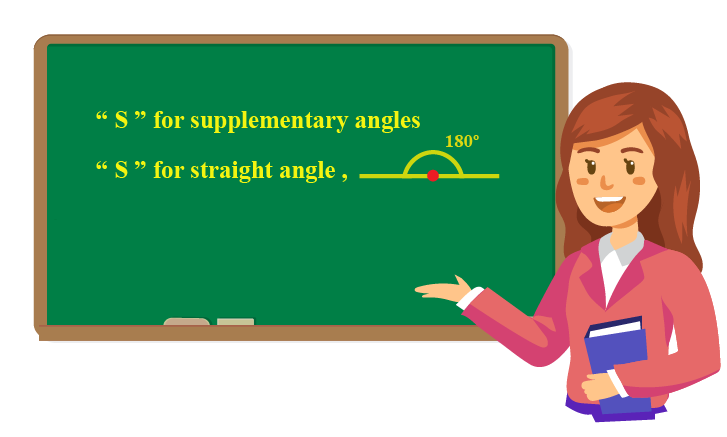## How to Find Supplement of an Angle? (With an Activity)

We know that the sum of two supplementary angles is 180 degrees and each of them is said to be a "Supplement" of each other.

Thus, the supplement of an angle is found by subtracting it from 180 degrees.

 Supplement of $$x^\circ$$ is $$(180-x)^\circ$$

Example:

What is the supplement of 77o?

Solution

The supplement of 77o is obtained by subtracting it from 180o.

Thus, its supplement is:

$(180-77)^\circ =103^\circ$

Here is an activity to check how well you have understood the method to find the supplement of an angle.

You can click and drag the "Orange" dot to change the angles and then you can enter the supplement of the given angle.

## Properties of Supplementary Angles

The properties of supplementary angles are as follows.

• Only two angles can be supplementary.

Even if the sum of three angles is 180 degrees, they are NOT considered supplementary.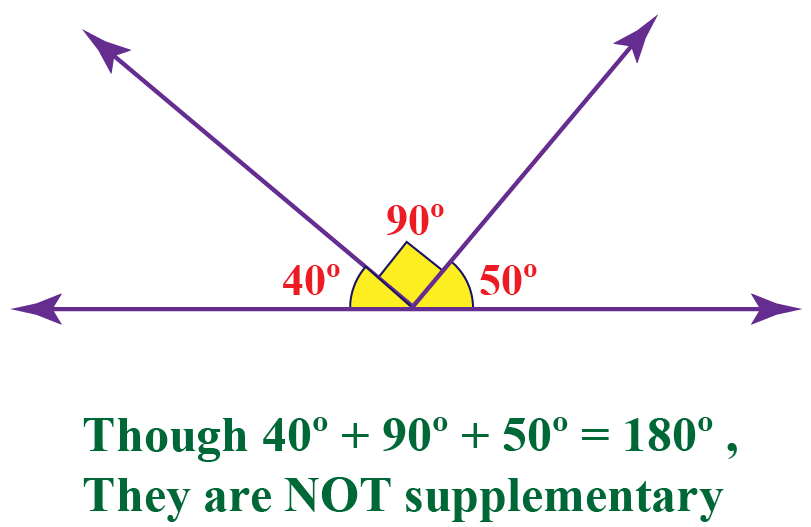• If two angles are supplementary, then either both of them are right angles or one of them is acute and one of them is obtuse.

In other words, both supplementary angles can be neither acute nor obtuse.

## Supplementary Angle Theorem (with Illustration)

The supplementary angle theorem states that "if two angles are supplementary to the same angle, then they are congruent to each other".

### Proof of Supplementary Angle Theorem

Consider the following figure: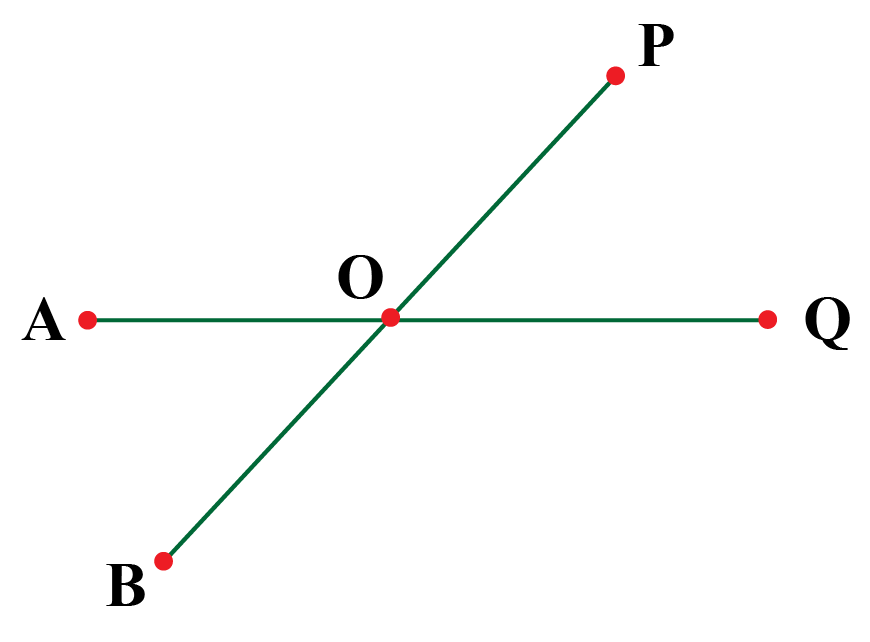Let us assume that $$\angle POQ$$ is supplementary to $$\angle AOP$$ and $$\angle BOQ$$.

Then by the definition of supplementary angles,

\begin{align} \angle POQ + \angle AOP &= 180^\circ\\[0.3cm] \angle POQ + \angle BOQ &=180^\circ \end{align} From the above two equations, we can say that $\angle POQ + \angle AOP=\angle POQ + \angle BOQ$ Subtracting $$\angle POQ$$ from both sides, $\angle AOP = \angle BOQ$ Hence, the theorem is proved.

You can visualize the supplementary angle theorem using the following illustration.

Help your child score higher with Cuemath’s proprietary FREE Diagnostic Test. Get access to detailed reports, customized learning plans, and a FREE counseling session. Attempt the test now.

## Solved Examples

 Example 1

Find angle $$Y$$ in the following figure.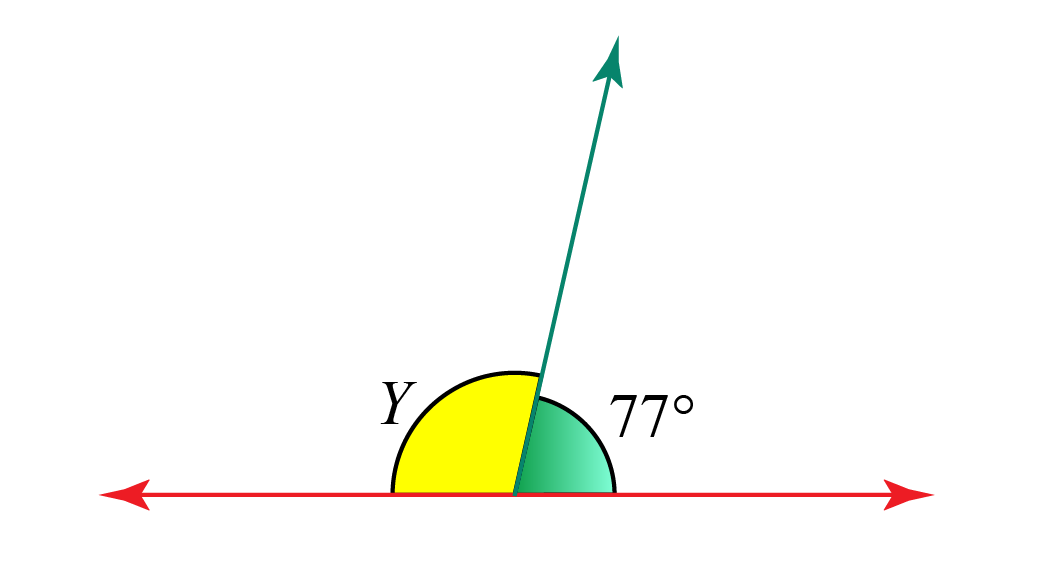Solution:

In the given figure, $$Y$$ and 77o are supplementary as they lie at a point on a straight line.

Hence, their sum is 180o.

\begin{align} Y +77^\circ &= 180^\circ \\[0.2cm] Y &= 180^\circ-77^\circ\\[0.2cm] Y &= 103^\circ \end{align}

 $$\therefore$$ $$Y=103^\circ$$
 Example 2

Find the values of $$\angle A$$ and $$\angle B$$, if $$\angle A$$ and $$\angle B$$ are supplementary such that $$\angle A=2x+10$$ and $$\angle B=6x−46$$.

Solution:

Since $$\angle A$$ and $$\angle B$$ are supplementary, their sum is 180o.

\begin{align} \angle A+\angle B &=180\\[0.2cm] (2x+10)+(6x-46)&=180\\[0.2cm] 8x - 36&=180\\[0.2cm] 8x&=216\\ x &= 27 \end{align}

Therefore, \begin{align} \angle A &= 2(27)+10 = 64^\circ\\[0.2cm] \angle B &= 6(27)-46 =116^\circ \end{align}

 $$\therefore$$ \begin{align} \angle A &= 64^\circ\0.2cm] \angle B & =116^\circ \end{align}  Example 3 Find the value of $$x$$ if the following two angles are supplementary.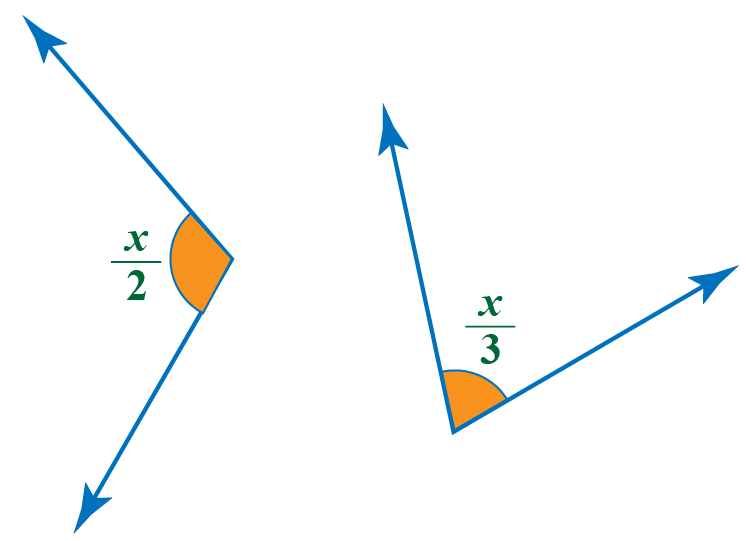Solution: Since the given two angles are supplementary, their sum is 180o. \[ \begin{align} \dfrac{x}{2}+ \dfrac{x}{3}&=180\\[0.2cm] \dfrac{5x}{6}&=180\\[0.2cm] x&=180 \times \dfrac{6}{5}\\[0.2cm] x &= 216\end{align}

 $$\therefore$$ $$x = 216$$
 Example 4

Two angles are supplementary.

The measure of the larger angle is 5 degrees more than 4 times the measure of the smaller angle.

What is the measure of the larger angle in degrees?

Solution:

Let us assume that the two supplementary angles are $$x$$ (larger) and $$y$$ (smaller).

By the given information,$x = 4y+5$

Since the two angles are supplementary, their sum is 180o

\begin{align} x+y&=180\\[0.2cm] (4y+5)+y &=180 & [\because x=4y+5]\\[0.2cm] 5y+5&=180\\[0.2cm] 5y&=175\\[0.2cm] y&=35 \end{align}

Thus, the larger angle is, $x = 4(35)+5=145^\circ$

 $$\therefore$$ Larger angle = $$145^\circ$$Challenging Questions
1. Find the value of $$a+b-2c$$ in the following figure.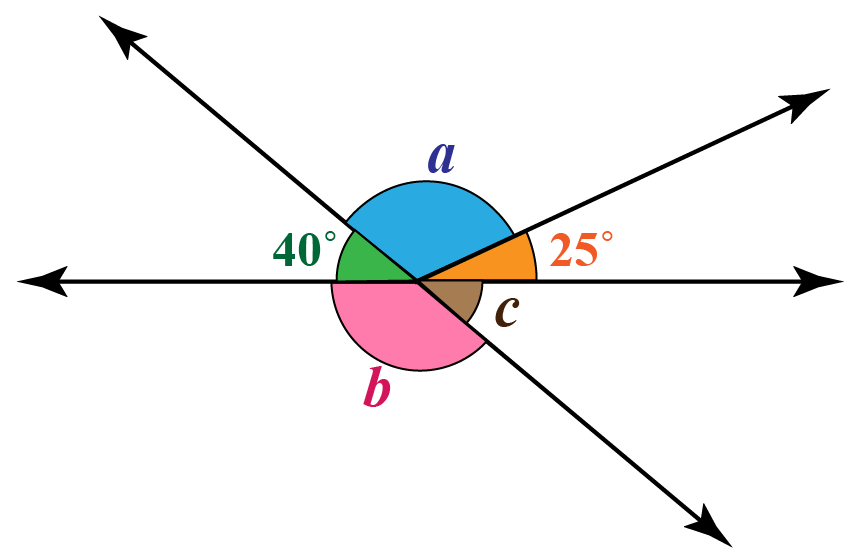CLUEless in Math? Check out how CUEMATH Teachers will explain Supplementary Angles to your kid using interactive simulations & worksheets so they never have to memorise anything in Math again!

Explore Cuemath Live, Interactive & Personalised Online Classes to make your kid a Math Expert. Book a FREE trial class today!

## Practice Questions

Here are a few problems.

IMO (International Maths Olympiad) is a competitive exam in Mathematics conducted annually for school students. It encourages children to develop their math solving skills from a competition perspective.

## 1. How do you find supplementary angles?

If the sum of two angles is 180 degrees, then we say that they are supplementary.

Thus, the supplement of an angle is obtained by subtracting it from 180

For example, the supplement of $$40^\circ$$ is $$180-40=140^\circ$$.

## 2. Can two acute angles be supplementary angles?

No, if two angles are supplementary then they are both either right angles or one of them is acute and one of them is obtuse.

## 3. Can three angles be supplementary?

No, three angles can never be supplementary.

The definition of supplementary angles holds true only for two angles.

## 4. Can two right angles be supplementary angles?

Yes, two right angles are always supplementary as they add up to 180 degrees.

More Important Topics
Numbers
Algebra
Geometry
Measurement
Money
Data
Trigonometry
Calculus